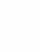• The Algorithm on how to Implement Gaussian Distribution for Anomaly Detection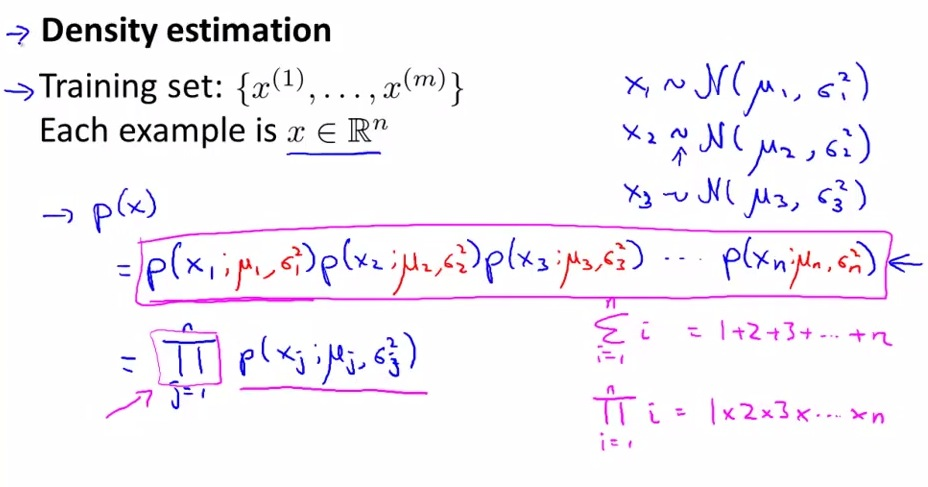• Now introduce a Density estimation, use for estimating where the p(x) on (epsilon) threshold value
• the x itself is matrix of vector. where x(i) is a vector of features. And each of x has each mew and sigma value
• funny symbol = product of each iteration
• Dense way for writing formula

•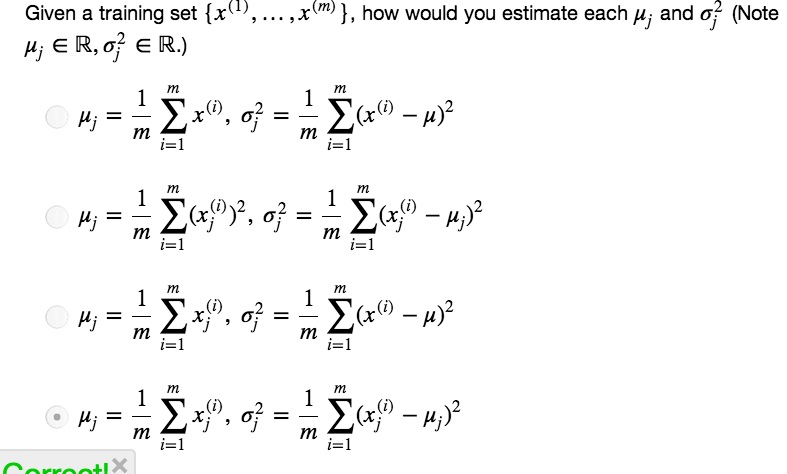•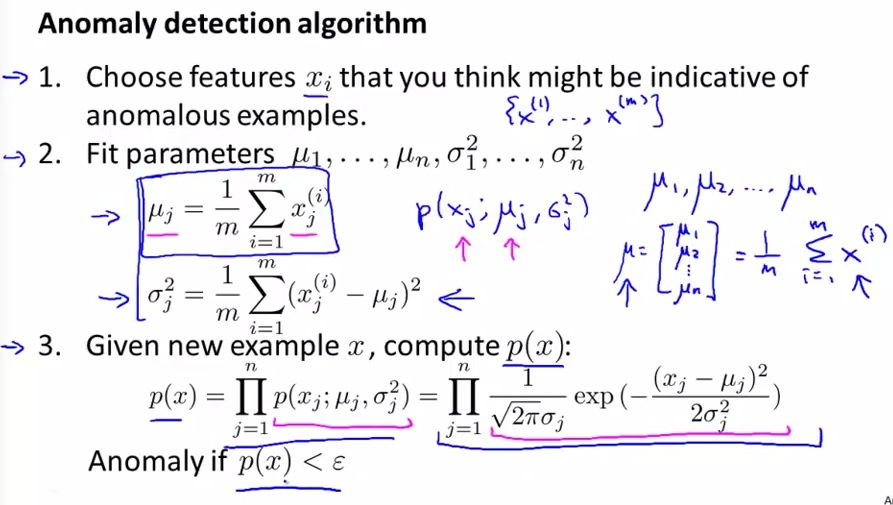• Choose which features that probably is the anomalous examples(too small or too big)
• Possible to produce vectorized implementation for mew and sigma
• According to step 3, compute p(x) and observe if it smaller than the threshold value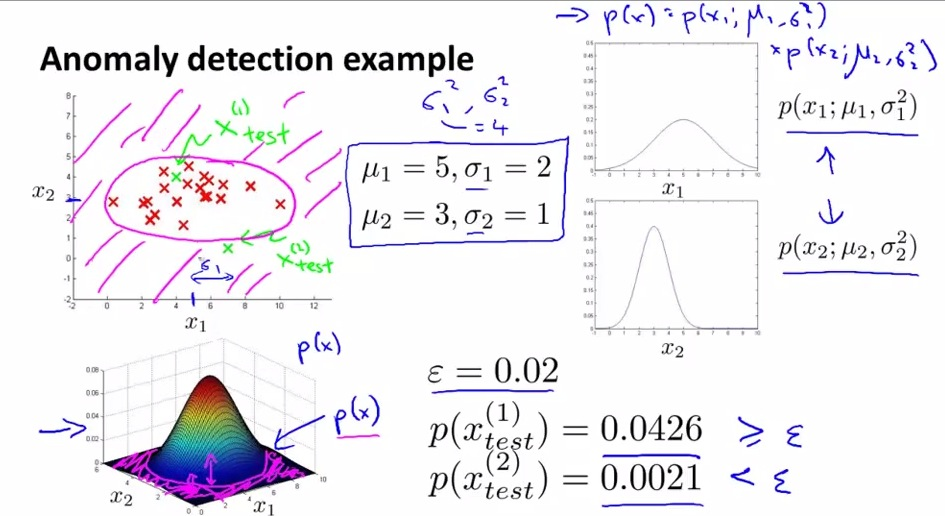• Set manually epsilon (discussed later how to automatically set)
• p(x1) based on result is not an anomaly, whereas p(x2) prove x2 is an anomaly
• all points outside the circle region is an anomaly
• As said before inside the circle is sure, outside is doubtly(anomaly).

• We now know how to  getting data set, taking mew and sigma into account and determining whether xi is the anomalous examples
• How to actually get this to work well in Anomaly Detection